## 3.12.2023

### Sine and cosine table

This is the most unusual table of sines and cosines. The idea to make such a table came to me in the process of writing these articles:

I strongly recommend that you read these articles. Then you will know how easy it is to calculate the values of sine and cosine yourself. I made one sine and cosine table from these three articles.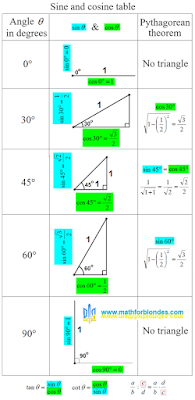Sine and cosine table

The table indicates which sides of the triangle to take as a unit. For 0 and 90 degrees, triangles do not exist, these are ordinary segments.

The third column of the table shows how to use the Pythagorean theorem to calculate the desired value of the sine or cosine. For a cosine of 30 degrees and a sine of 60 degrees, the calculations are the same.

At the bottom of the table there are hints on how to calculate the tangent and cotangent values if you know the sine and cosine values. If you forgot how to divide one fraction by another, use one more hint. You need to multiply the first fraction by the reciprocal of the second fraction.

## 3.11.2023

### Sine and cosine of 45 degrees

We have already considered what the sine of 30 degrees is and what the sine of 60 degrees is equal to. Now we will see how to find the sine and cosine of 45 degrees and why they are equal.

Last time we considered an equilateral triangle. Now we will consider an isosceles right triangle. A very rare beast in a herd of triangles. Mathematicians have known it for many thousands of years, and it is simply boring for mathematicians to tinker with it.

## Isosceles right triangle

We will consider a tired isosceles right triangle. Why is the triangle tired? He lay down on his side to rest.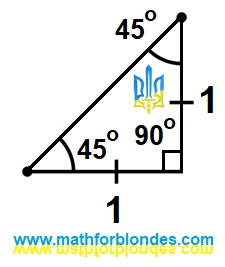Isosceles right triangle

The legs of this triangle are equal to one. Once again I repeat that both legs have the same length. We do not know the length of the hypotenuse of this triangle, but we can easily calculate it using the Pythagorean theorem.

## Sine and cosine of 45 degrees

Smart people came to us and said that the sine and cosine of an angle are equal to the ratio of the legs to the hypotenuse. Since the legs of a right triangle with an angle of 45 degrees are equal, then the value of the sine of 45 degrees is equal to the value of the cosine of 45 degrees. We take the math in hand and calculate this value.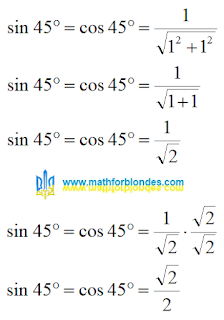Sine and cosine of 45 degrees

Why did I multiply the numerator and denominator of a fraction by the square root of two? Small children do not like lumps in porridge. Mathematicians don't like square roots in denominators. Very capricious uncles and aunts.

## 3.03.2023

### What is the sine of 60 degrees?

Before we look for the answer to the question: "What is the sine of 60 degrees?", I strongly recommend reading the post "Why is the sine of 30 degrees equal to half one?".

## Height of an equilateral triangle

I again take an equilateral triangle with a side equal to one. I draw height.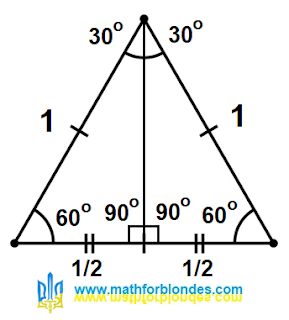Height of an equilateral triangle

After that, I will not turn anything. I'll just remove half of the drawing.

## Cosine 60 degrees

I have a right-angled triangle, the diagonal of which is equal to one.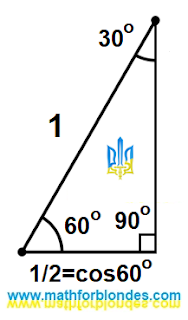Cosine 60 degrees

The base of this triangle is equal to 1/2 and is also equal to the cosine of an angle of 60 degrees.

## Sine 60 degrees

The height of this triangle is equal to the sine of the angle of 60 degrees. We calculate the height using the Pythagorean theorem.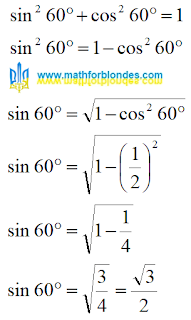Sine 60 degrees

The sine of 60 degrees is equal to the square root of three divided by two. It is calculated in the same way as the cosine of 30 degrees.